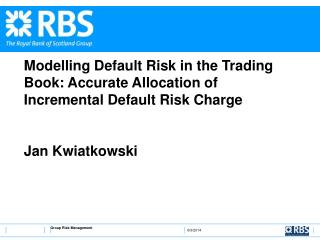# Modelling Default Risk in the Trading Book: Accurate Allocation of Incremental Default Risk Charge Jan Kwiatkowski - PowerPoint PPT PresentationDownload PresentationModelling Default Risk in the Trading Book: Accurate Allocation of Incremental Default Risk Charge Jan Kwiatkowski

Modelling Default Risk in the Trading Book: Accurate Allocation of Incremental Default Risk Charge Jan KwiatkowskiDownload Presentation## Modelling Default Risk in the Trading Book: Accurate Allocation of Incremental Default Risk Charge Jan Kwiatkowski

- - - - - - - - - - - - - - - - - - - - - - - - - - - E N D - - - - - - - - - - - - - - - - - - - - - - - - - - -
##### Presentation Transcript

1. Modelling Default Risk in the Trading Book: Accurate Allocation of Incremental Default Risk ChargeJan Kwiatkowski Group Risk Management

2. Summary • Background on IDRC • Trading book default risk models • The need for accurate allocation • Andersen, Sidenius & Basu (ASB) algorithm • Conditional Expected Loss; an allocation metric • Calculation using Bayes’ Theorem • Extensions

3. Background on IDRC • Required to find high percentile of portfolio default losses over a given time horizon. • We prefer Conditional Expected Shortfall (CES) at equivalent percentile: • The book tends to be ‘lumpy’; therefore we must include idiosyncratic effects.

4. Trading book default risk models(calibrated to a given time-horizon) • Many models use systematic risk factors and correlations

5. Conditional PDs • Require an algorithm for computing distribution of portfolio loss conditional on any X • Integrate over X (e.g. Monte Carlo or quadrature)

6. Algorithms for conditional losses • Monte Carlo • Transforms • ASB • Must keep in mind the need for allocation

7. Allocation of IDRC • Total IDRC is allocated/attributed to contributors (down to position level), and aggregated up the organisational hierarchy. • Allocation must be ‘fair’ and consistent • Especially, desks with identical positions should get the same allocation. • Using Monte Carlo for high percentiles , we are at the mercy of relatively few random numbers • Transforms not convenient for allocation

8. The ASB algorithm Discretise LGD’s as multiples of a fixed ‘Loss Unit’ ui= loss units for issuer i Let qi= PD for issuer i (conditional on givenX ) Recursively compute the distribution of the losses for portfolios consisting of the first i exposures only, for i =0, 1, 2, …., N • The method is exact modulo discretisation • Parcell (2006) shows how effects of discretisation may be mitigated • Easily extended to multiple outcomes

9. ASB implementation

10. A metric for allocation Exactly accounts for portfolio CES

11. Bayes’ Theorem

12. Allocation methodology • We can easily calculate this by removing issuer i fromthe final portfolio and adding its LGD, ui, to the resulting portfolio distribution. • We use the reversal of the ASB algorithm to remove issuer i

13. Reversal of ASB We illustrate this for a long position (ui>0); this is easily adaptable to short positions.

14. Warning • This becomes unstable forqi>0 close to 1. • Can be mitigated (Parcell 2006)

15. Summary of method – Phase 1 • For various systematic effects, X, use ASB to find the conditional distribution. • Integrate over X • Compute Lα, the required portfolio CES

16. Summary of method – Phase 2 • For each issuer, i: • for each X • Use reverse ASB to find the distribution with i defaulted. • Compute the corresponding probability that Lα is exceeded • Multiply by uiqi/(1-α) • Integrate over X

17. Possible Extensions • The method for VaR (rather than CES) is even simpler • Multiple outcomes: • Stochastic LGDs • Rating Downgrades • Also upgrades, but requires matrix inversion • Structured products – cascade structure